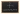Coordinate Planes: Practice Problems
POSTED ON APRIL 15, 2020## Becoming Familiar with the Coordinate Plane

In this pre-algebra lesson, Juni Mathematics instructor Genesis will be teaching you about the coordinate plane and key terms to know.

Coordinate planes are important to understand because they help us know how to read graphs, understand points in space, and even apply concepts in other subjects like data science and coding!

Read Genesis' Intro to Coordinate Planes lesson first to understand what coordinate planes are and how to use them. Then, use what you've learned with the warmup questions on this page to practice drawing coordinate planes and mapping points and lines.

Once you've checked your answers below, you can also keep practicing with Genesis' Drills for Segments in a Coordinate Plane (coming soon).

## Warmup Problems

1. Draw a coordinate plane that has an x-axis that runs from -6 to 6 and a y-axis that runs from -7 to 7.
2. Draw a coordinate plane that has an x-axis that runs from -5 to 5 and a y-axis that also runs from -5 to 5. Then draw a point, A, at the coordinates (-1, 4).
3. Draw a coordinate plane that has an x-axis that runs from -3 to 5 and a y-axis that runs from -3 to 5. Then draw a point, B, at the coordinates (3, 2).
4. What are the coordinates of the midpoint C on segment AB?5. What are the coordinates of the midpoint O on segment MN?6. Are the points A and B collinear?7. Are the points F and G collinear?Find solutions and Genesis' tutorial video below!

## Solutions Video

Watch Instructor Genesis walk through every problem solution.

### Juni Instructor Genesis' Tutorial:

1.2.3.4. (6, -2)
5. (-2, 4)
6. Yes
7. No

## More Practice for Coordinate Planes

We hope you enjoyed Genesis' Warmup Problems! Continue practicing coordinate planes with the drills below. Or, review key terms and concepts with Genesis' Intro to Coordinate Planes lesson and video.

## Need help or want to keep learning?

We hope you enjoyed Genesis' Lesson on Points, Lines, and Coordinate Planes! This lesson falls under our Pre-Algebra B course curriculum.

To keep practicing or learning, please check out all of our math and coding tutorials on our Home Learning Resources page.

Need help? Looking up your questions is one of the best ways to learn! Another great way to learn is from an experienced math instructor. From elemenatary math to calculus, Juni offers online math classes to accelerate your child's learning.